# Working with database using Pandas

Performing various operations on data saved in SQL might lead to performing very complex queries that are not easy to write. So to make this task easier it is often useful to do the job using pandas which are specially built for data preprocessing and is more simple and user-friendly than SQL.

There might be cases when sometimes the data is stored in SQL and we want to fetch that data from SQL in python and then perform operations using pandas. So let’s see how we can interact with SQL databases using pandas.

This is the database we are going to work with
diabetes_data

Note: Assuming that we the data is stored in sqlite3

 `# import the libraries ` `import` `sqlite3 ` `import` `pandas as pd ` ` `  `# create a connection ` `con ``=` `sqlite3.connect(``'Diabetes.db'``) ` ` `  `# read data from SQL to pandas dataframe. ` `data ``=` `pd.read_sql_query(``'Select * from Diabetes;'``, con) ` ` `  `# show top 5 rows ` `data.head() `

Output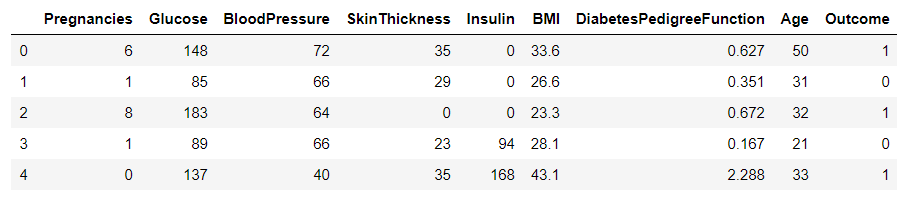## Basic operation

• Slicing of rows
We can perform slicing operations to get the desired number of rows from within a given range.
With the help of slicing, we can perform various operations only on the specific subset of the data

 `# read the data from sql to pandas dataframe. ` `data ``=` `pd.read_sql_query(``'Select * from Diabetes;'``, con) ` ` `  `# slicing the number of rows  ` `df1 ``=` `data[``10``:``15``] ` `df1 `

Output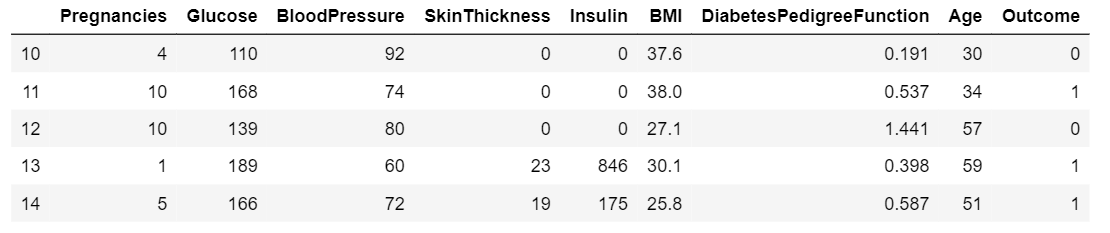• Selecting specific columns
To select a particular column or to select number of columns from the dataframe for further processing of data.

 `# read the data from sql to ` `# pandas dataframe. ` `data ``=` `pd.read_sql_query(``'Select * from Diabetes;'``, con) ` ` `  `# selecting specific columns. ` `df2 ``=` `data.loc[:, [``'Glucose'``, ``'BloodPressure'``]].head() ` `df2 `

Output: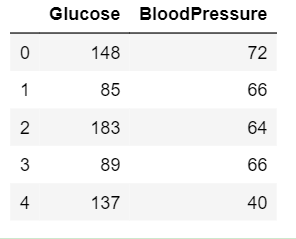• Summarize the data
In order to get insights from data, we must have a statistical summary of data. To display a statistical summary of the data such as mean, median, mode, std etc. We perform the following operation

 `# read the data from sql  ` `# to pandas dataframe. ` `data ``=` `pd.read_sql_query(``'Select * from Diabetes;'``, con) ` ` `  `# summarize the data ` `data.describe() `

Output: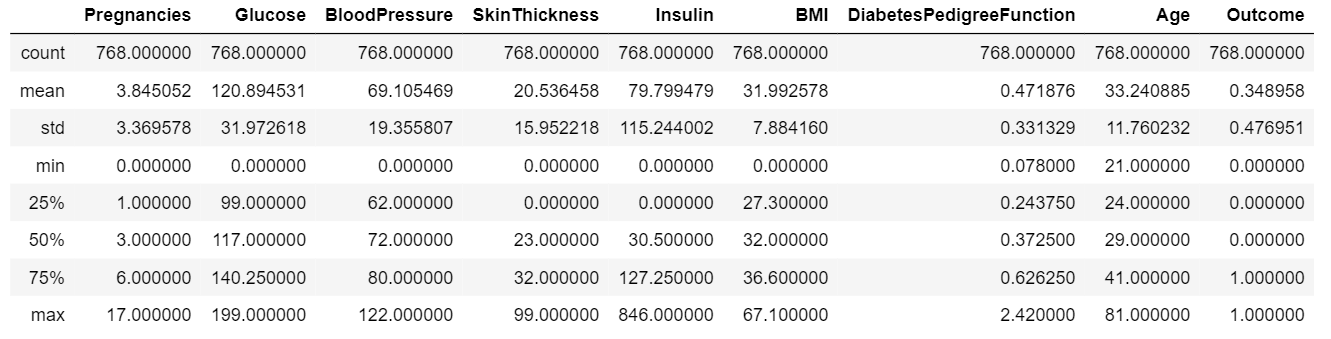• Sort data with respect to a column
For sorting the dataframe with respect to a given column values

 `# read the data from sql  ` `# to pandas dataframe. ` `data ``=` `pd.read_sql_query(``'Select * from Diabetes;'``, con) ` ` `  `# sort data with respect  ` `# to particular column. ` `data.sort_values(by ``=``'Age'``).head() `

Output: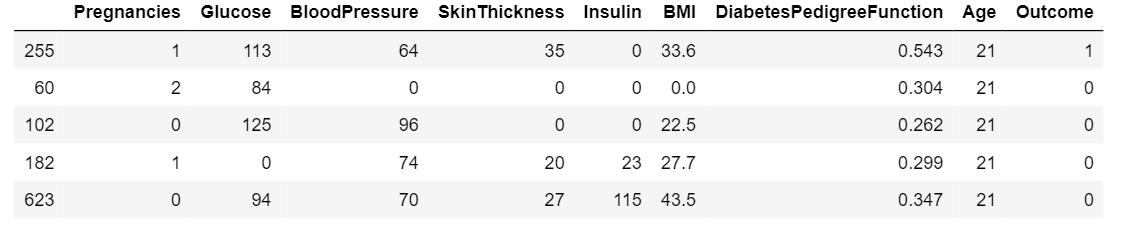• Display mean of each column
To Display the mean of every column of the dataframe.

 `# read the data from sql  ` `# to pandas dataframe. ` `data ``=` `pd.read_sql_query(``'Select * from Diabetes;'``, con) ` ` `  `# count number of rows and columns ` `data.mean() `

Output: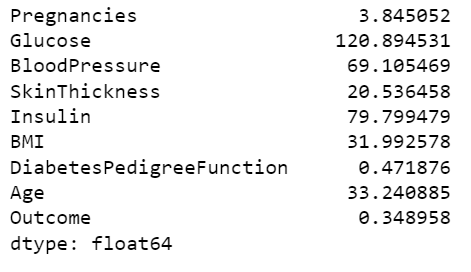My Personal Notes arrow_drop_upCheck out this Author's contributed articles.

If you like GeeksforGeeks and would like to contribute, you can also write an article using contribute.geeksforgeeks.org or mail your article to contribute@geeksforgeeks.org. See your article appearing on the GeeksforGeeks main page and help other Geeks.

Please Improve this article if you find anything incorrect by clicking on the "Improve Article" button below.

Improved By : Akanksha_Rai

Article Tags :

Be the First to upvote.

Please write to us at contribute@geeksforgeeks.org to report any issue with the above content.